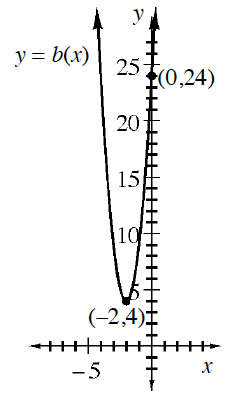### Home > INT2 > Chapter 9 > Lesson 9.4.1 > Problem9-133

9-133.

Consider each function below.

1.      $x$

$a(x)$

$0$

$10$

$1$

$10.5$

$2$

$11.025$

$3$

$11.576$

$4$

$12.155$

$5$

$12.763$

1.1. Describe each function completely.

(i) is exponential. Use the starting value and multiplier to write the equation.
(ii) is a quadratic. Use the vertex and the stretch factor to write the equation.

$a(x)$ is an increasing exponential function with a growth rate of $5\%$ or a multiplier of $1.05$.
The equation is $a(x) = 10(1.05)^x$
$b(x)$
is a quadratic function with vertex $(-2, 4)$ and a vertical stretch factor of $5$. It is increasing for $x > -2$ and has $y$-intercept $(0, 24)$
The equation is $b(x) = 5(x + 2)^2 + 4$

2. Sketch a graph of each function on the same set of axes. Based on what you know about quadratic and exponential growth, what will eventually happen to the graphs of the functions as $x$ gets large?

Eventually $a(x)$ will intersect $b(x)$ and then exceed it.